Home > MC2 > Chapter 11 > Lesson 11.3.1 > Problem11-88

11-88.

Multiple Choice: Which of the cylinders matches the net? Explain how you know. Note that the length above each cylinder is the radius of the base. Homework Help ✎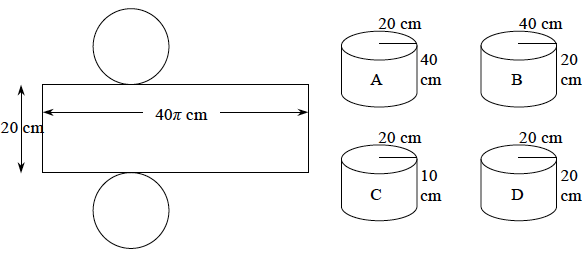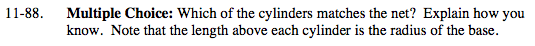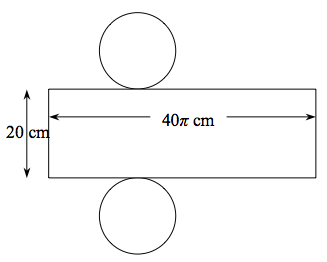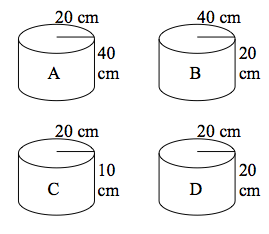Refer to the Math Notes from Lesson 10.2.6 for help.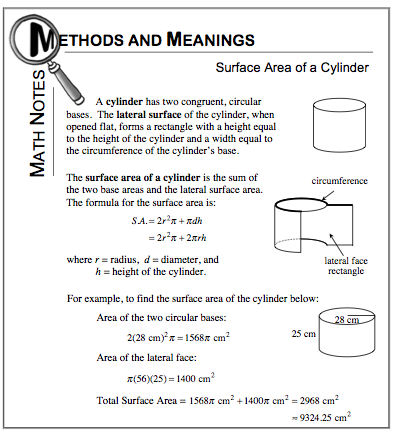Notice that the height of the lateral face rectangle is the same as the height
for the cylinder.

That means the cylinder must have a height of 20 cm, which eliminates
both A and C.

From the Math Notes box you can see that the circumference of the base
is the width of the lateral face rectangle. Which cylinder has a
circumference of 40? cm?

Since circumference=(diameter)(π) we need to know which cylinder has a diameter
of 40 cm. How can you find the diameter?

Diameter is twice the radius, so the radius must be 20 for the diameter to be 40.
The answer is cylinder D.